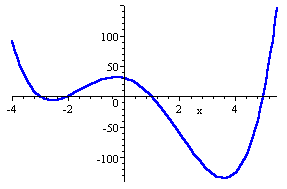Finding Solutions

In the study of polynomial equations, it is important to understand how to find the solution of the equation. Equations of higher degree allow for many solutions. The maximum number of solutions possible is equal to the degree of the polynomial. Graphs can be used to find the solutions of polynomial equations.

When a polynomial equation is set equal to 0, the solutions of the polynomial are the x-intercepts or roots of the corresponding graph. For example, to solve the equation, x4 ? x3 ? 19x2 ? 11x + 31 = 0 graphically, means to find the roots or x-intercepts of the corresponding function, f(x) = x4 ? x3 ? 19x2 ? 11x + 31. The graph below shows that there are 4 roots, at approximately x = -3, x = -2, x = 1, and x = 5. While these are not exact answers, they can be used to find exact answers using a table.Listen